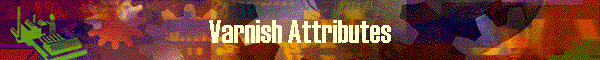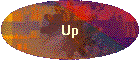This table defines the equation to calculate weight of varnish.

The entry is to calculate the varnish weight per square inch.   DynaRod will try to calculate the entire surface area of any cross section rod according to this table.

So far, the first table entry is only used since the weight of varnish does not make difference among the kinds of varnish.   The factors in the equation comes from the experiment of measuring several samples.

Data on Screen

 Item Meaning No record number Type Type of varnish Varnish K The value of factor K of equation y = K x + C    x is dimension Varnish C The value of constant C of equation y = K x + C    x is dimension Remarks comments